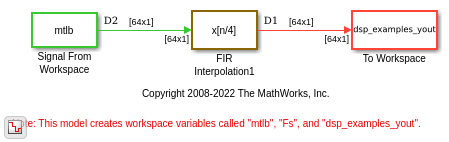# FIR Interpolation Using Multirate Frame-Based Processing

In this example, the FIR Interpolation block interpolates the signal by increasing the sample rate of the signal, while maintaining the frame size.

Open the model. The input to the FIR Interpolation block is a single-channel input with a frame size of 64 and a frame period of 1 second. In the block dialog box, set the Interpolation factor parameter to 4 and the Rate options parameter to `Allow multirate processing`.

Run the model. The block operates in the multirate frame-based processing mode. The input and output signals of the FIR Interpolation block have the same frame size, but the sample rate of the output is L times the sample rate of the input, where L is the interpolation factor. With an interpolation factor of 4, the sample rate of the output signal is 4 times the sample rate of the input signal. The color coding in the model shows the sample time (inverse of sample rate) of the signal at both the input and output ports of the FIR Interpolation block.